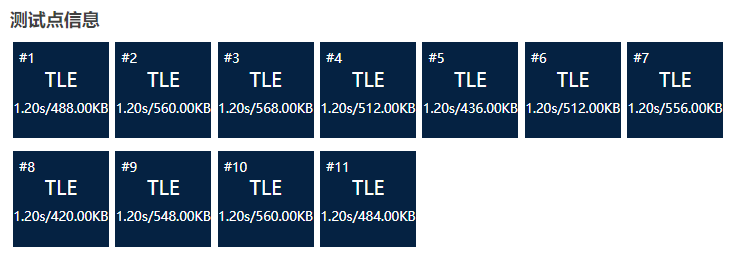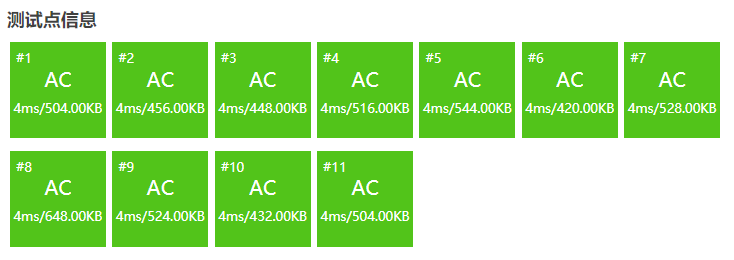﻿ P2181 刷题记录

### P2181 刷题记录

``````#include <stdio.h>

int factorial(int n) {
int result;
if(n == 0 || n == 1) return 1;
else {
result = factorial(n);
return result;
}
}

int C(int n){
int r = n - 4;
int up = factorial(n);
int down = factorial(r) * factorial(n-r);
int result = up / down;
return result;
}

// C(r, n) = n!/[r!(n-r)!]
// C(n-4, n)

int main() {
int n;
scanf("%d", &n);
int result = C(n);
printf("%d", result);

return 0;
}
``````

``````#include <stdio.h>

unsigned long long n, ans;

int main() {
scanf("%lld", &n);
ans = n * (n - 1) / 2 * (n - 2) / 3 * (n - 3) / 4;
printf("%lld
", ans);

return 0;
}
``````一个计算凸多边形对角线交点的方法[J]沈文德.蘇州教育學院學報.1996
 ww3113306 P2181題解 包括公式推導過程